Register for Maths, Science, English, Reasoning Olympiad Exams Register here | Book Free Demo for Live Olympiad Classes here | Check Olympiad Exam Dates here | Buy Practice Papers for IMO, IOM, HEO, IOEL etc here | Login here to participate in all India free mock test on every Saturday

# Number System Integers and Rational Numbers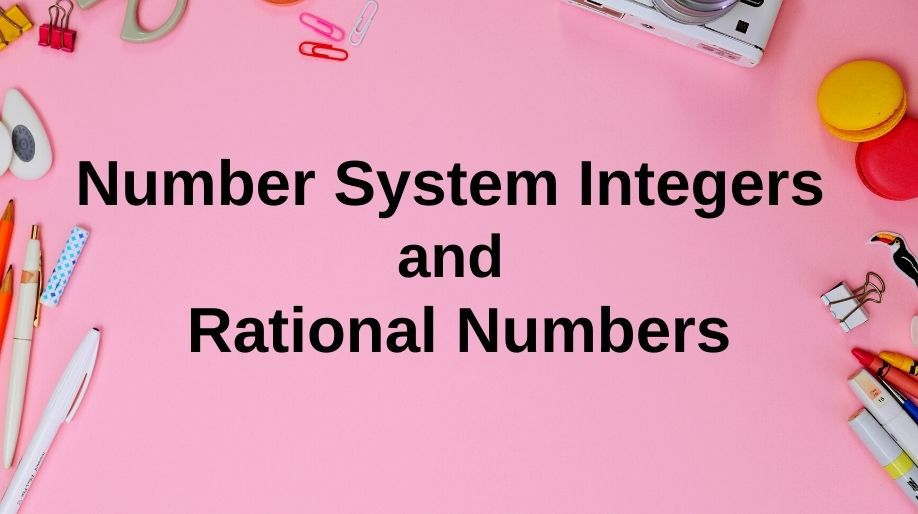To add two rational numbers, first express each rational number with a positive denominator.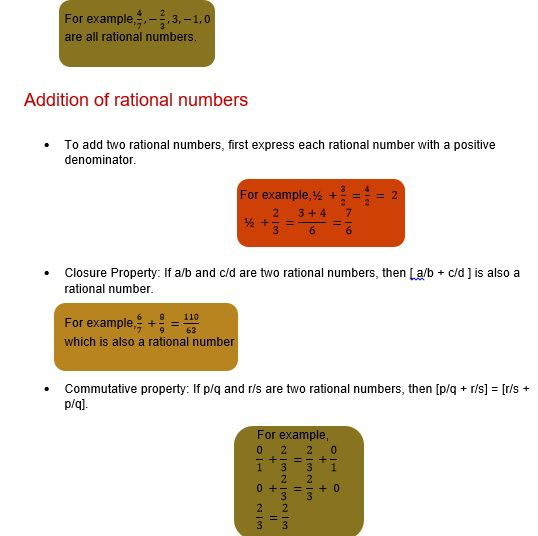•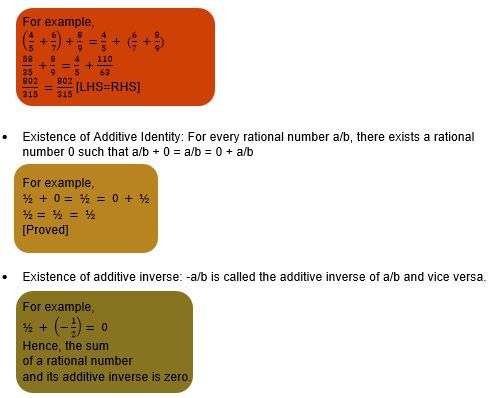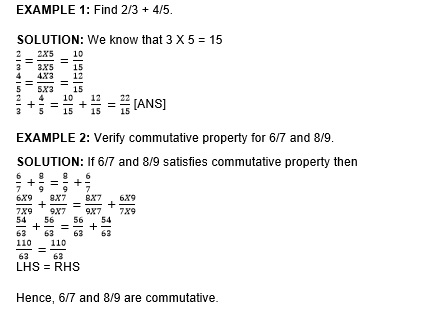# Subtraction of rational numbers

Subtracting one rational number from another rational number is same as adding the additive inverse(negative) of the rational number that is being subtracted to the other rational number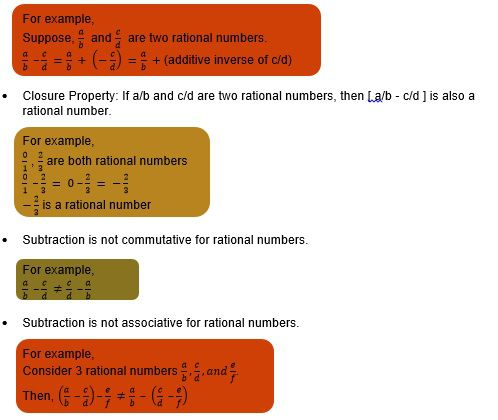EXAMPLE 1: Sum of two rational number is 1/6. If one of them is -1/2, then find the other rational number.

SOLUTION: The other rational number is = 1/6 – (-1/2) =1/6 + ½ = 2/3 [ANS]

EXAMPLE 2: What number should be added to -1/4 to get -4/5?

SOLUTION: The number is = -4/5 – (-1/4) = -4/5 + ¼ = -11/20 [ANS]

Multiplication of rational number

•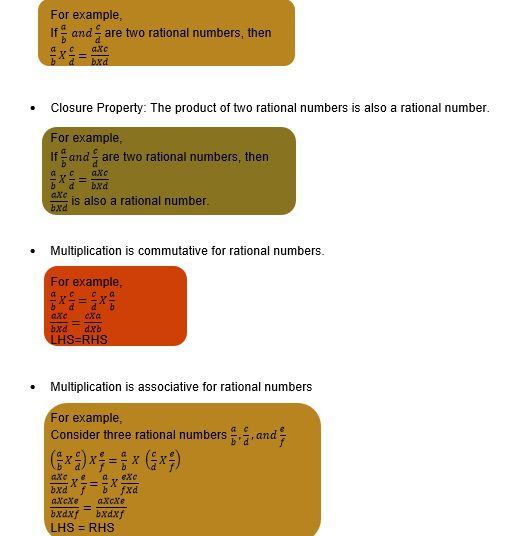•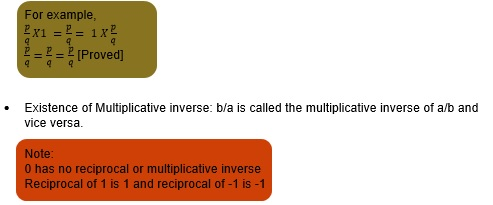•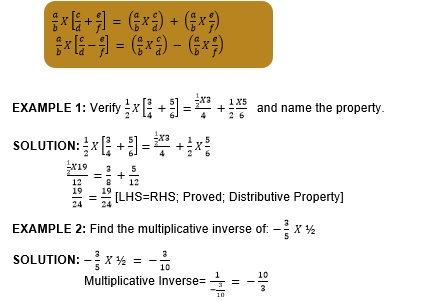•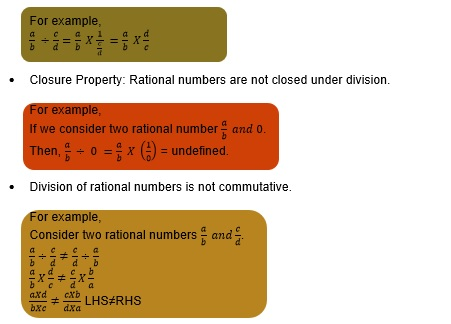•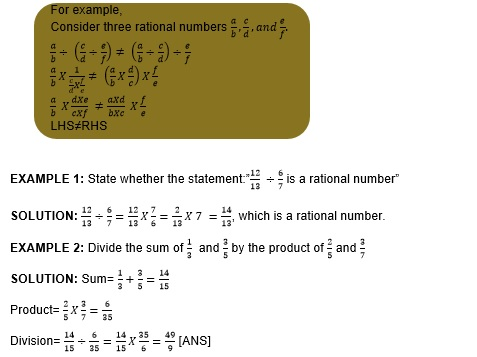# Representation of a rational number on a number line

To represent rational number on a number line proceed as under: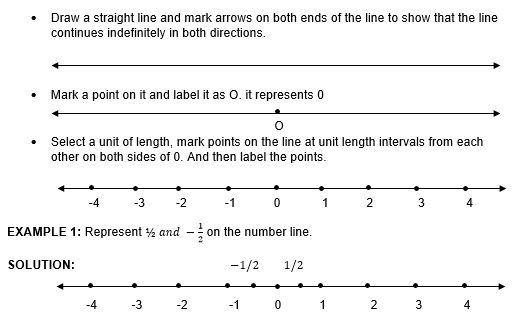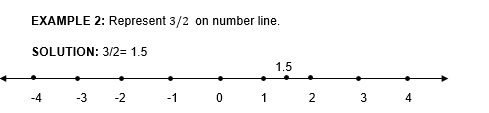# Rational numbers between two rational numbers

To insert n rational numbers between two rational numbers, do the following:

• When given rational numbers have the same denominator multiply the denominator and numerator by (n+1).
• When given rational numbers have different denominators: find the LCM of the denominators; change the given rational number into equivalent rational numbers with LCM as their denominator; If necessary multiply the denominator and numerator of each by (n+1).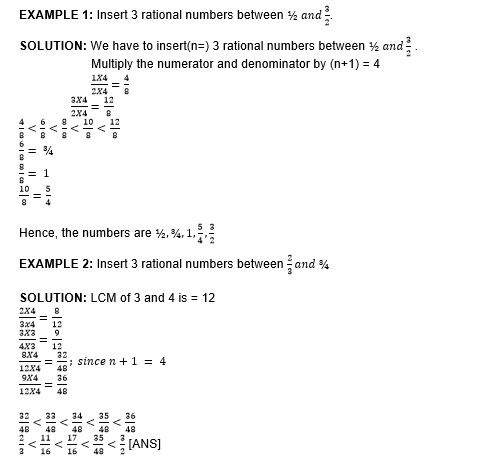# Practice these questions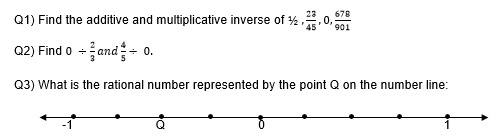Q4) Vivan donated of his salary to the orphanage, 1/4th of his salary on food, 1/3 of his salary on rent and electricity, and 1/15th of his salary on telephone bills. This month he donated Rs. 6000 in a charity. He is left with Rs. 4000. Find his monthly salary.

Q5) Area of a square is 1 sq. metre more than ½ of the area of a rectangle. If the area of the square is 91 sq. metre. Then find the dimensions of the rectangle. Given breadth is ¾ times of length.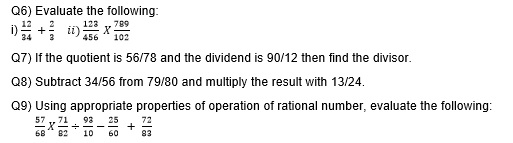# Recap

• All integers and fractions are real numbers
• Addition is closed, commutative, associative.
• Multiplication is closed, commutative, associative and distributive

Division is not closed, not commutative, not associative.

## Quiz for Number System Integers and Rational Numbers

 Q.1 The additive inverse of any integer a is _____________. a) 0 b) a c) 1 d) -a
 Q.2 Complete the pattern: –11, – 8, – 5, – 2, _______ a) 1 b) 0 c) -1 d) 2
 Q.3 Fill in the box to make the statements true.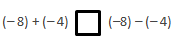a) = b) < c) > d) none of the above
 Q.4 Identify an irrational number. a) √144 b) √24 c) 2.5 d) 2/8
 Q.5 Complete the pattern 7, 3, – 1, – 5,______ a) 8 b) -8 c) -9 d) 10
 Q.6 Find the equivalent of following rational numbers (-99)/3 a) 33/(-1) b) (-33)/1 c) 1/33 d) (-1)/33
 Q.7 Find the equivalent of following rational numbers 5/8 a) 15/40 b) 5/24 - 5/6 c) 9/8 - 1/2 d) none of the above
 Q.8 Find the equivalent of following rational numbers 13/6 a) 8/3 + 1/2 b) 8/3 - 2/1 c) 78/6 d) none of the above
 Q.9 Fill in the box to make the statements true.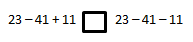a) > b) < c) ≤ d) ≥
 Q.10 Which of the following is an irrational number? a) 2.393939… b) 2.0 c) 2.101001000… d) 2.778